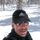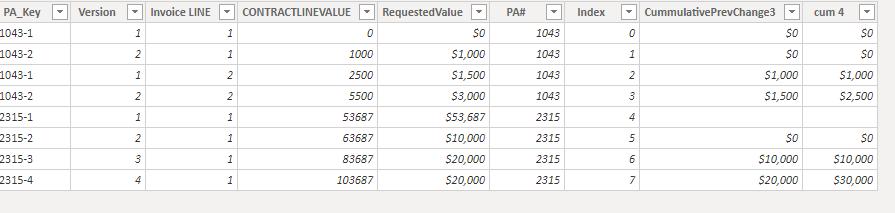cancel
Showing results for
Did you mean:

Fabric is Generally Available. Browse Fabric Presentations. Work towards your Fabric certification with the Cloud Skills Challenge.Anonymous
Not applicable

## Cummulative Prev Change

Good afternoon

I am struggling with a DAX formula, and will appreciate some help

Want to create a formula for "Cummulative Prev Change" as per screenshot and following conditions

1) First two rows have to be zero

2) from 3rd row, the formula should get PreviousVersions's "Requested Value" + PreviousVersions's "Cummulative Prev Change" if

I tried following formula, to no effect 😞

CummulativePrevChange2 = IF(PA_KEY[Version]>1,LOOKUPVALUE(PA_KEY[RequestedValue],PA_KEY[Version],PA_KEY[Version]-1,PA_KEY[PA#],PA_KEY[PA#]),0)

Attached are the sample file and screenshot of how the column should calculate values...

3 ACCEPTED SOLUTIONSSuper User

Hi @Anonymous
The solution at this point is two columns.

Let me know if you have any questions.

If this solves your issues, please mark it as the solution, so that others can find it easily. Kudos are nice too.
Nathaniel

``````Cumulative =

var _curIndex =PA_KEY[Index]
var _minIndex = CALCULATE(MIN(PA_KEY[Index]),ALLEXCEPT(PA_KEY,PA_KEY[PA#]),PA_KEY[Index]<_curIndex)

return

IF(_curIndex = _minIndex || _curIndex = _minIndex+1,BLANK(), CALCULATE(sum(PA_KEY[RequestedValue]),ALLEXCEPT(PA_KEY,PA_KEY[PA#]),PA_KEY[Index]=_curIndex -1))``````

``````Cumulative and previous =
VAR _curIndex = PA_KEY[Index]   //Get current Index from this row
VAR _minIndex = CALCULATE ( MIN ( PA_KEY[Index] ), ALLEXCEPT ( PA_KEY, PA_KEY[PA#] ), PA_KEY[Index] < _curIndex )    //Get minimum index for this PA#

VAR _prev3 = CALCULATE ( SUM ( PA_KEY[Cumulative] ),  ALLEXCEPT ( PA_KEY, PA_KEY[PA#] ), PA_KEY[Index] = _curIndex - 1 )   // Get the previous row amount from the Cumulative column

RETURN
IF (                                        //Return a blank if the current index is equal to the first two rows of this PA# else do the calc and add the previous row from the Cumulative column
_curIndex = _minIndex
|| _curIndex = _minIndex + 1,
BLANK (),
CALCULATE (
SUM ( PA_KEY[RequestedValue] ),
ALLEXCEPT (
PA_KEY,
PA_KEY[PA#]
),
PA_KEY[Index] = _curIndex - 1
) + _prev3
)``````

Proud to be a Super User!Super User

@Anonymous

Just this portion is the measure and it relies on the index column @Nathaniel_C  added to your sample table.

``````Column =
VAR _MinIndex = CALCULATE ( MIN ( PA_KEY[Index] ), ALLEXCEPT ( PA_KEY, PA_KEY[PA#] ) )
VAR _RowIndex = [Index]
RETURN CALCULATE ( SUM ( PA_KEY[RequestedValue] ), ALLEXCEPT ( PA_KEY, PA_KEY[PA#] ), PA_KEY[Index] > _MinIndex && PA_KEY[Index] < _RowIndex )``````

Are you able to add an index column to your source data that will properly order the lines?Super User

The problem with using the original table is you don't know what order the lines are going to come in unless you give it an order by.  You could add the index on the SQL side as well, something like this.

``````SELECT
PA_Key,
Version,
[Invoice LINE],
CONTRACTLINEVALUE,
REQUESTEDVALUE,
PA#,
ROW_NUMBER() OVER( ORDER BY ( SELECT 0 ) ) AS RowIndex
FROM Table
ORDER BY
PA_Key,
Version,
[Invoice LINE]``````

10 REPLIES 10Super UserProud to be a Super User!Anonymous
Not applicable

thank you Nathan,

Kindly can you share the DAX for your column?

thank you

-UsmanSuper User

Hi @Anonymous
The solution at this point is two columns.

Let me know if you have any questions.

If this solves your issues, please mark it as the solution, so that others can find it easily. Kudos are nice too.
Nathaniel

``````Cumulative =

var _curIndex =PA_KEY[Index]
var _minIndex = CALCULATE(MIN(PA_KEY[Index]),ALLEXCEPT(PA_KEY,PA_KEY[PA#]),PA_KEY[Index]<_curIndex)

return

IF(_curIndex = _minIndex || _curIndex = _minIndex+1,BLANK(), CALCULATE(sum(PA_KEY[RequestedValue]),ALLEXCEPT(PA_KEY,PA_KEY[PA#]),PA_KEY[Index]=_curIndex -1))``````

``````Cumulative and previous =
VAR _curIndex = PA_KEY[Index]   //Get current Index from this row
VAR _minIndex = CALCULATE ( MIN ( PA_KEY[Index] ), ALLEXCEPT ( PA_KEY, PA_KEY[PA#] ), PA_KEY[Index] < _curIndex )    //Get minimum index for this PA#

VAR _prev3 = CALCULATE ( SUM ( PA_KEY[Cumulative] ),  ALLEXCEPT ( PA_KEY, PA_KEY[PA#] ), PA_KEY[Index] = _curIndex - 1 )   // Get the previous row amount from the Cumulative column

RETURN
IF (                                        //Return a blank if the current index is equal to the first two rows of this PA# else do the calc and add the previous row from the Cumulative column
_curIndex = _minIndex
|| _curIndex = _minIndex + 1,
BLANK (),
CALCULATE (
SUM ( PA_KEY[RequestedValue] ),
ALLEXCEPT (
PA_KEY,
PA_KEY[PA#]
),
PA_KEY[Index] = _curIndex - 1
) + _prev3
)``````

Proud to be a Super User!Super User

Hi @Anonymous , @jdbuchanan71 ,
You are in luck! This is from @jdbuchanan71 , one column and much simpler code.

``````Hi Nathaniel,

The problem I see is how to determine the order of the lines.  I know you added an index but will the rows always come out of the source in the right order?  I would verify that with the original poster.

If the index can be added safely then this code should work.

Column =
VAR _MinIndex = CALCULATE ( MIN ( PA_KEY[Index] ), ALLEXCEPT ( PA_KEY, PA_KEY[PA#] ) )
VAR _RowIndex = [Index]
RETURN CALCULATE ( SUM ( PA_KEY[RequestedValue] ), ALLEXCEPT ( PA_KEY, PA_KEY[PA#] ), PA_KEY[Index] > _MinIndex && PA_KEY[Index] < _RowIndex )

=========================

We don't need to do any checking for the first 2 rows because the filters  PA_KEY[Index] > _MinIndex && PA_KEY[Index] < _RowIndex take care of it.

On index line 5 for example.

_MinIndex = 4

_RowIndex = 5

We ask for the sum of PA_KEY[RequestedValue] where the Index is > 4 AND < 5.  No such lines so we get nothing.
On Index line 7 we as for the sum of [RequestedValue] where the Index > 4 AND < 7.  We get the sum of Index 5 and 6``````

Nathaniel

Proud to be a Super User!Super User

@Anonymous

Just this portion is the measure and it relies on the index column @Nathaniel_C  added to your sample table.

``````Column =
VAR _MinIndex = CALCULATE ( MIN ( PA_KEY[Index] ), ALLEXCEPT ( PA_KEY, PA_KEY[PA#] ) )
VAR _RowIndex = [Index]
RETURN CALCULATE ( SUM ( PA_KEY[RequestedValue] ), ALLEXCEPT ( PA_KEY, PA_KEY[PA#] ), PA_KEY[Index] > _MinIndex && PA_KEY[Index] < _RowIndex )``````

Are you able to add an index column to your source data that will properly order the lines?Anonymous
Not applicable

thank you JDBuchanon, and Nathan for your replies

Adding an index (order by) will make query quite slow.

thank you again

-UsmanSuper User

@Anonymous

If the data always come into the model in the correct order you can just add an index row in the query editor the way @Nathaniel_C did.

Without an index column, what it the logic to determine "previous row"?  Keep in mind that PowerBI does not understand "this row is the row before the row I am on" the way excel does.  That is why we need an index column or some other logic to tell PowerBI how to search the entire table and find the previous rows.  Also, what is your data source for the information?Anonymous
Not applicable

"PA_Key" and "Invoice Line" is the unique key/index; e.g. "1043-1-1" which shows "Purchase Order-Version#-Invoice Line#" respectively

Can we somehow use the original table to do it, or would we have to add a new index and use order by clause, to always order them by Purchase Order, Invoice Line#, Version # ?

thank youSuper User

The problem with using the original table is you don't know what order the lines are going to come in unless you give it an order by.  You could add the index on the SQL side as well, something like this.

``````SELECT
PA_Key,
Version,
[Invoice LINE],
CONTRACTLINEVALUE,
REQUESTEDVALUE,
PA#,
ROW_NUMBER() OVER( ORDER BY ( SELECT 0 ) ) AS RowIndex
FROM Table
ORDER BY
PA_Key,
Version,
[Invoice LINE]``````Anonymous
Not applicable

Thank you JDBuchanan and Nathan, for all you help.

Much appreciated 🙂Announcements#### Power BI Monthly Update - November 2023

Check out the November 2023 Power BI update to learn about new features.#### The largest Power BI and Fabric virtual conference

130+ sessions, 130+ speakers, Product managers, MVPs, and experts. All about Power BI and Fabric. Attend online or watch the recordings.Top Solution Authors
Top Kudoed Authors
Users online (5,367)